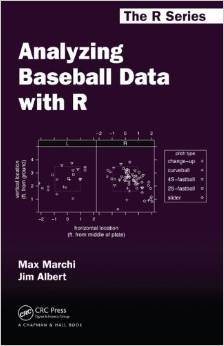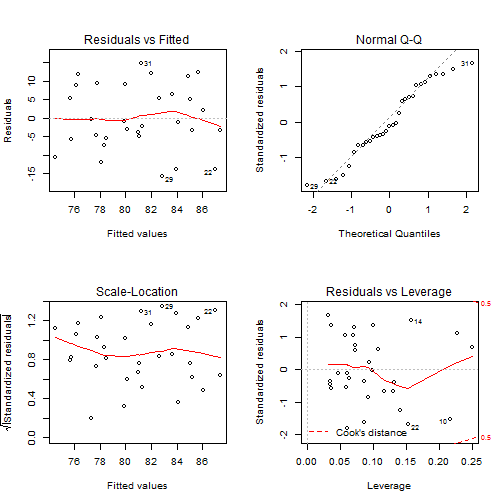# Data Analysis IV: Multi-Variate Regression

SE350 Team

### Introducing Multi-variate Regression

Multi-variate regression works in exactly the same way that simple regression works, with the exception that it is using more than one explanatory variable. This also means that it is not in two dimensional space, and is harder to picture mentally, although all the same ideas apply.

The basic equation for multi-variate regression is

$y=\beta_0+\beta_1 x_1+\beta_2 x_2+\dots+\beta_m x_m+e$

### Choosing Variables

• Ideally pick values that you can justify based on practical or theoretical grounds
• You could also choose variables that generate the largest value of Adjusted $$R^2$$, or
• You could choose those with the most significant p-values
• Let the computer choose the best variables for you

# Remember, when looking at the goodness-of-fit for multi-variate regression models, you must use adjusted $$R^2$$!

### Note that R is often the tool of choice for baseball data:### Multi-variate Regression with Baseball Data

We will import Baseball TEAM data:

team<-read.csv("BaseballTeam2014.csv",as.is=TRUE)


Note: This is a new data set that was not provided in the original data bundle

### Team Glossary (1 of 3)

Name Description
Tm Team
W Wins
L Losses
X.Bat Number of Players used in Games
BatAge Batters’ average age
R.G Runs Scored Per Game
G Games Played or Pitched
PA Plate Appearances
AB At Bats
R Runs Scored/Allowed
H Hits/Hits Allowed

### Team Glossary (2 of 3)

Name Description
X2B Doubles Hit/Allowed
X3B Triples Hit/Allowed
HR Home Runs Hit/Allowed
RBI Runs Batted In
SB Stolen Bases
CS Caught Stealing
BB Bases on Balls/Walks
SO Strikeouts
BA Hits/At Bats
OBP (H + BB + HBP)/(At Bats + BB + HBP + SF)

### Team Glossary (3 of 3)

Name Description
SLG Total Bases/At Bats or (1B + 2*2B + 3*3B + 4*HR)/AB
OPS. On-Base + Slugging Percentages
OPS+ OPS+100*[OBP/lg OBP + SLG/lg SLG - 1] Adjusted to the player’s ballpark(s)
TB Total Bases
GDP Double Plays Grounded Into
HBP Times Hit by a Pitch.
SH Sacrifice Hits (Sacrifice Bunts)
SF Sacrifice Flies
IBB Intentional Bases on Balls
LOB Runners Left On Base

### First Multi-variate Regression:

First, let's create a column for the Win-Loss percentage, and then select six variables that would theoretically most contribute to successful teams:

team$WLperc<-team$W/(team$W+team$L)
team.lm<-lm(W~R.G+R+H+HR+RBI+OBP,data=team)


### Summary of Model:

summary(team.lm)


Call:
lm(formula = W ~ R.G + R + H + HR + RBI + OBP, data = team)

Residuals:
Min     1Q Median     3Q    Max
-15.43  -5.67  -1.40   7.88  15.79

Coefficients:
Estimate Std. Error t value Pr(>|t|)
(Intercept) -1.38e+01   7.78e+01   -0.18    0.861
R.G         -3.06e+02   8.20e+02   -0.37    0.712
R            1.97e+00   5.07e+00    0.39    0.700
H           -6.68e-02   5.22e-02   -1.28    0.213
HR          -2.57e-03   1.13e-01   -0.02    0.982
RBI         -1.10e-01   3.03e-01   -0.36    0.719
OBP          6.37e+02   3.56e+02    1.79    0.086 .
---
Signif. codes:  0 '***' 0.001 '**' 0.01 '*' 0.05 '.' 0.1 ' ' 1

Residual standard error: 9.64 on 24 degrees of freedom
Multiple R-squared:  0.164, Adjusted R-squared:  -0.0445
F-statistic: 0.787 on 6 and 24 DF,  p-value: 0.589


### Dropping Variables (HR):

• Remember that this model and p-values are heavily influenced by all of the other variables in the model.
• You have to drop variables one at a time (starting with the variable with the highest p-value)
team.lm<-lm(W~R.G+R+H+RBI+OBP,data=team)  ##Drop HR


### New Summary:

summary(team.lm)


Call:
lm(formula = W ~ R.G + R + H + RBI + OBP, data = team)

Residuals:
Min     1Q Median     3Q    Max
-15.48  -5.68  -1.41   7.87  15.79

Coefficients:
Estimate Std. Error t value Pr(>|t|)
(Intercept)  -14.6840    65.6724   -0.22    0.825
R.G         -306.6092   802.9815   -0.38    0.706
R              1.9805     4.9575    0.40    0.693
H             -0.0667     0.0507   -1.31    0.201
RBI           -0.1141     0.2512   -0.45    0.654
OBP          641.1294   310.0744    2.07    0.049 *
---
Signif. codes:  0 '***' 0.001 '**' 0.01 '*' 0.05 '.' 0.1 ' ' 1

Residual standard error: 9.45 on 25 degrees of freedom
Multiple R-squared:  0.164, Adjusted R-squared:  -0.00269
F-statistic: 0.984 on 5 and 25 DF,  p-value: 0.447


### Dropping Variables (R.G):

Try one more variable. The highest new p-value is R.G.

team.lm<-lm(W~R+H+RBI+OBP,data=team)  ##Drop R.G


### New Summary:

summary(team.lm)


Call:
lm(formula = W ~ R + H + RBI + OBP, data = team)

Residuals:
Min     1Q Median     3Q    Max
-15.28  -4.99  -2.35   7.96  14.75

Coefficients:
Estimate Std. Error t value Pr(>|t|)
(Intercept) -12.0270    64.2210   -0.19    0.853
R             0.0899     0.2405    0.37    0.712
H            -0.0720     0.0479   -1.50    0.145
RBI          -0.1067     0.2463   -0.43    0.668
OBP         638.6336   304.8705    2.09    0.046 *
---
Signif. codes:  0 '***' 0.001 '**' 0.01 '*' 0.05 '.' 0.1 ' ' 1

Residual standard error: 9.29 on 26 degrees of freedom
Multiple R-squared:  0.16,  Adjusted R-squared:  0.0302
F-statistic: 1.23 on 4 and 26 DF,  p-value: 0.321


### Now let the computer do it for you

The function we will use to do this is stepAIC. This function uses the Akaike information criterion (AIC) to measure the relative quality of a statistical model. AIC handles the trade-off between the goodness of fit of a model and the complexity of the model. The stepAIC function is found in the MASS package in R. The MASS package ships with R, so you don't have to install it. It does not automatically load when you start R, however, and so you need to call library(MASS) before using the stepAIC function.

library(MASS)
##Build Original Model
team.lm<-lm(W~R.G+R+H+HR+RBI+OBP,data=team)
winner<-stepAIC(team.lm)


### Summary of Winning Model:

summary(winner)


Call:
lm(formula = W ~ H + OBP, data = team)

Residuals:
Min     1Q Median     3Q    Max
-15.82  -5.30  -1.12   7.62  14.77

Coefficients:
Estimate Std. Error t value Pr(>|t|)
(Intercept)   0.2436    52.6178    0.00    0.996
H            -0.0784     0.0385   -2.04    0.051 .
OBP         603.9873   273.8446    2.21    0.036 *
---
Signif. codes:  0 '***' 0.001 '**' 0.01 '*' 0.05 '.' 0.1 ' ' 1

Residual standard error: 8.99 on 28 degrees of freedom
Multiple R-squared:  0.152, Adjusted R-squared:  0.0919
F-statistic: 2.52 on 2 and 28 DF,  p-value: 0.0987


### Checking Assumptions:

Just like last lesson, we once again need to check our four regression assumptions. Remember that our assumptions were:

1. The relationship between the explanatory variables and the response variable is linear.
2. Errors in prediction of the value of Y are distributed in a way that approaches the normal curve.
3. Errors in prediction of the value of Y are all independent of one another. This means that all of the explanatory variables are independent of one another.
4. The distribution of the errors in prediction of the value of Y is constant regardless of the value of X (constant variance).

### Checking Linearity, Equal Variance, Normality, and Outliers: